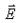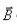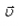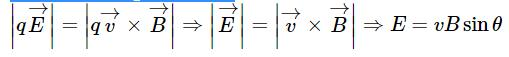Courses

# Test: Motion In Combined E And B Fields

## 5 Questions MCQ Test Physics For JEE | Test: Motion In Combined E And B Fields

Description
This mock test of Test: Motion In Combined E And B Fields for JEE helps you for every JEE entrance exam. This contains 5 Multiple Choice Questions for JEE Test: Motion In Combined E And B Fields (mcq) to study with solutions a complete question bank. The solved questions answers in this Test: Motion In Combined E And B Fields quiz give you a good mix of easy questions and tough questions. JEE students definitely take this Test: Motion In Combined E And B Fields exercise for a better result in the exam. You can find other Test: Motion In Combined E And B Fields extra questions, long questions & short questions for JEE on EduRev as well by searching above.
QUESTION: 1

### Ratio of magnetic force Fm and electric force Fe acting on a charge getting undeflected through the field is

Solution:

if the particle is undeflected, then the magnetic force equals the electric force:
q x v x B=q x E
B is the magnitude of the magnetic field
E is the magnitude of the electric field
v is the magnitude of the velocity of the particle.
If the forces are equal then:
v=E/B
For undeflected v=1
E/B=1

QUESTION: 2

### Time taken by an ion to describe a semicircular path, in a cyclotron

Solution:

We also know for a circular motion time period = 2πm/qb as the charge in question has. Constant q, mass is also constant, b is also constant thus time period for semicircular path is constant.

QUESTION: 3

### If a charged particle goes undeflected in a region containing electric and magnetic fields. Select all of the following statements that must be true for this. 1.must be perpendicular to2.must be perpendicular to3.must be perpendicular to4.  E must be equal to vB​

Solution:

If a charged particle goes unaccelerated in a region containing
electric and magnetic fields,QUESTION: 4

The dipole moment of a current loop is independent of:

Solution:

Dipole moment of a current loop
M=N ×I × A
So, it is dependent on current in the loop, number of turns and area of the loop.
It is independent of the magnetic field in which it is situated.

QUESTION: 5

If cathode rays are projected at right angles to a magnetic field, their trajectory is

Solution:

The magnetic force acts perpendicular to the velocity of the particle. This  causes circular motion. In the magnetic field the speed and kinetic energy of the particle remain constant, but the direction is altered at each instant by the perpendicular magnetic force.